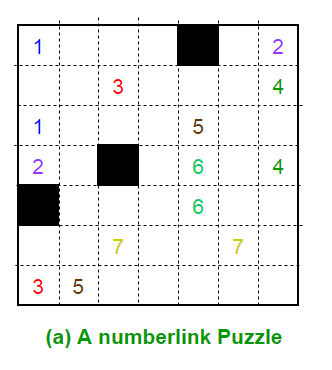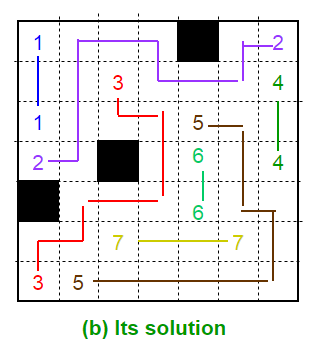# A Number Link Game

• Difficulty Level : Easy
• Last Updated : 09 Feb, 2018

The Game : Consider an n × n array of squares. Some of the squares are empty, some are solid, and some non-solid squares are marked by integers 1, 2, 3, … Each integer occupies exactly two different squares on the board. The task of the player is to connect the two occurrences of each integer on the board by a simple path using horizontal and vertical movements alone. No two different paths are allowed to intersect one another. No path may include any solid square (solid squares are forbidden to appear on any path). Finally, all non-solid squares must be filled by the paths.

The Algorithm : To prepare a valid random puzzle with a given board size n × n, we first generate random simple mutually non-intersecting paths on the board. If a few isolated squares remain outside all the generated paths, mark these isolated squares as solid (forbidden). We then supply the endpoints of the paths and the list of the solid squares as the puzzle.
Thus we first generate a solution, and then work out the puzzle from the solution. The paths and the solid squares partition the n × n board. We use a union-find data structure to generate this partition. The data structure deals with the subsets of the set of n^2 squares on the board.PseudoCode:

1. Locate squares (i, j) and (k, l) randomly on the board such that:
(a) (i, j) and (k, l) are neighbors of one another, and
(b) neither (i, j) nor (k, l) belongs to any path generated so far. If no such pair of squares is found on the entire board, return FAILURE     /* Here, (i, j) and (k, l) are the first two squares on the new path to be constructed. */
2. Make a union of the two union-find trees containing (i, j) and (k, l).
3. Repeat so long as the current path can be extended:
Rename (i, j) = (k, l).
Locate a random neighboring square (k, l) of (i, j) such that:
(a) (k, l) does not belong to any path generated so far (including the current one)
(b) the only neighbor (k, l) has on the partially constructed current path is (i, j).
4. If no such neighbor (k, l) can be found, the path cannot be extended further, so break the loop
5. Otherwise, make the union of the two union-find trees to which (i, j) and (k, l) belong.
6. Set the endpoint flags of the two squares that are at the beginning and at the end of the new path.
7. Return SUCCESS

This article is contributed by Vaibhav Aggarwal. If you like GeeksforGeeks and would like to contribute, you can also write an article and mail your article to contribute@geeksforgeeks.org. See your article appearing on the GeeksforGeeks main page and help other Geeks.

Please write comments if you find anything incorrect, or you want to share more information about the topic discussed above

My Personal Notes arrow_drop_up